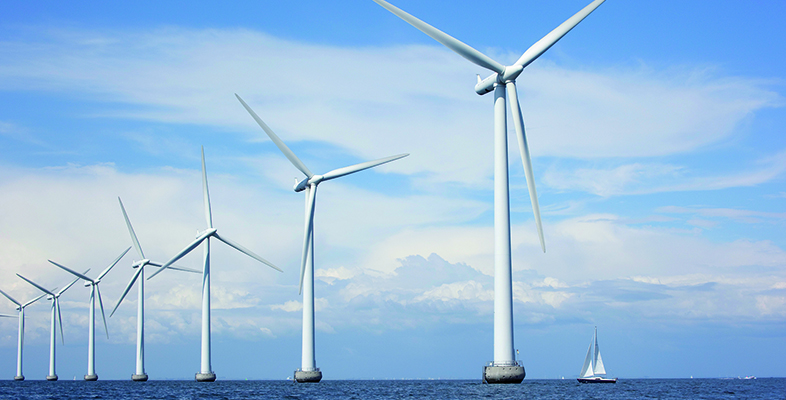Nature & Environment

### Become an OU studentEnergy in buildings

Start this free course now. Just create an account and sign in. Enrol and complete the course for a free statement of participation or digital badge if available.

# 3.5 Heating systems and CO2 emissions

In assessing any particular building we are likely to be interested in its total fuel consumption and its CO2 emissions. The previous sections have given some guidance on estimating the likely net space heating demand. Adding an allowance for water heating, the total annual fuel use for heating can thus be calculated as:

• annual fuel use = annual heat demand / heating system thermal efficiency

Not all heating fuels produce the same amount of CO2 for a given amount of heat produced. Table 11 gives the emission factors for the main UK heating fuels. Note that natural gas is considerably better than heating oil or coal.

Table 11 CO2 emission factors for heating fuels
Emissions CO2 /

kg kWh–1

Natural gas 0.21
Liquefied petroleum gas (LPG) 0.24
Heating oil (gas oil) 0.30
House coal 0.40
Electricity (2020 generation mix) 0.18
(Source: BRE, 2022; BEIS, 2018a)

The figures are from SAP 10 (BRE, 2022) and they may not agree exactly with those from other sources.

Back in 1960, when UK electricity generation was almost all coal-fuelled, producing a kilowatt hour of electricity involved the emission of 1 kg of CO2. Increased use of nuclear power and gas fired generation reduced this figure down to about 0.5 kg kWh-1 in 1998 where it remained until 2013. Since then, the rising proportion of electricity from renewable sources (particularly wind power) has reduced the figure further to only 0.18 kg kWh-1 in 2020 and it is likely to fall even further in the future.

Using these emission figures and likely heating system efficiencies we can calculate the total annual CO2 production.

As described at the beginning of Section 3 the thermal efficiency of a heating system = useful heat output / fuel input.

So annual fuel use = useful heat demand / thermal efficiency

annual CO2 production = annual fuel use × CO2 emission factor

Consider, for example, a house with a total estimated net space heating demand of 10 000 kWh yr–1 plus a water heating demand of 5000 kWh yr–1.

What are its likely annual CO2 emissions if it is heated (a) by a condensing natural gas boiler with a thermal efficiency of 90% or (b) using off-peak storage heating with an assumed efficiency of 100% and a 2020 CO2 emission factor?

• a.Using a condensing gas boiler:
• total annual fuel use = (10 000 + 5000)/0.90 = 16 667 kWh yr–1
• CO2 emission factor = 0.21 kg kWh-1
• CO2 emissions = 16 667 × 0.21 = 3500 kg yr–1

• b.Using off-peak electric storage heaters:
• total annual fuel use = (10 000 + 5000) / 1.0 = 15 000 kWh yr–1
• electricity CO2 emission factor = 0.18 kg kWh-1
• annual CO2 emissions = 15 000 × 0.18 = 2700 kg yr–1

Thus, the electric heating option produces about 20% less CO2 than the gas heating. If we had used the higher 1998 emission factor for electricity, then the answer would have been completely different, with the electric heating option producing more than twice as much CO2 as gas heating. This change has had a major impact on the past policy of switching from electricity to gas as a way of reducing overall CO2 emissions.

## Activity 14

Supposing the house described above is in a rural location and doesn’t have access to mains natural gas. What are the likely annual CO2 emissions if it is heated:

• a.by a condensing liquefied petroleum gas (LPG) boiler with a thermal efficiency of 93%?
• b.by a ground source heat pump with a system performance factor (SPF) of 2.80?

Which has the lower annual CO2 emissions?

• a.Using a LPG condensing gas boiler:

total annual fuel use = (10 000 + 5000) / 0.93 = 16 129 kWh yr-1

From Table 11, the emission factor for LPG is 0.24 kg CO2 per kWh

So annual CO2 emissions = 16 129 × 0.24 = 3871 kg yr-1

• b.Using a heat pump:

The useful heat produced by the heat pump = SPF × electricity used.

So electricity used = useful heat / SPF

= (10 000 + 5000) / 2.80 = 5357 kWh yr-1

Annual CO2 emissions = 5357 × 0.18 = 964 kg yr-1

The heat pump wins with only one quarter of the CO2 emissions!